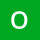Home
IT Knowledge
Inspiration
Languages
EN

# Python - create multiple subfolders

0 points
Created by:OneCricketer
340

In this article, we would like to show you how to create multiple subfolders in Python.

Quick solution:

``````import os

N = 10

for i in range(N):
os.makedirs(os.path.join("C:\\some_path\example_directory", "subfolder" + str(i)), exist_ok=True)``````

Note:

The `exist_ok` parameter was added in Python 3.5. Set it on `True` so you won't get `FileExistsError` if the directory exists.

## Practical example

In this example, we create a directory with multiple (`N`) subfolders in our project directory using `makedirs()` method from `os` module.

``````import os

N = 5

for i in range(N):
os.makedirs(os.path.join("example_directory", "subfolder" + str(i)), exist_ok=True)``````

if you want to create multiple subfolders outside the project folder, you need to specify the full path:

``````import os

N = 5

for i in range(N):
os.makedirs(os.path.join("C:\\some_path\example_directory", "subfolder" + str(i)), exist_ok=True)``````

result: